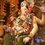# Proof of 0! = 1

0! = 1 can be proved using many methods. While some use patterns or logic, giving reasons for mathematical consistency & this & that, I shall use calculus here.

We have the gamma function denoted by $\Gamma(x)$, which is defined by

$\Gamma(x) = \int_0^\infty \mathrm{t}^{x-1}{e}^{-t}\,\mathrm{d}t$

This function arose while solving an interpolation problem. The problem was to find a monotonic function defined over $(1, \infty)$ which took the value $n!$ at $n$. It can be solved by evaluating the above improper integral which converges for $x>0$.

The gamma function is defined for the whole of real line provided we take $\Gamma(x)=\infty$ for $x=0,-1,-2,\dots$.

We are interested in the case when $x=n$ that is, a positive integer.

Integrating by parts, we have $\Gamma(n) = (n-1) \int_0^\infty \mathrm{t}^{(n-1)-1}{e}^{-t}\,\mathrm{d}t$

Notice that the integral is nothing but $\Gamma(n-1)$. Thus we have, $\Gamma(n)= (n-1) \times \Gamma(n-1)$.

Repeating the above process, $\Gamma(n)= (n-1)(n-2)(n-3) \dots\dots 1 \times \Gamma(1)$

But, $\Gamma(1) = \int_0^\infty \mathrm{t}^{x-1}{e}^{-t}\,\mathrm{d}t$ $=\left.\frac{e^{-t}}{-1}\right|_0^{\infty}$ $=-(0-1) = 1$

So, $\Gamma(n) = (n-1)!$

This must imply that $\Gamma(1) = 0!$ and we have already proved that $\Gamma(1)=1$. Thus, $0!=1$Note by Ameya Salankar
5 years, 8 months ago

This discussion board is a place to discuss our Daily Challenges and the math and science related to those challenges. Explanations are more than just a solution — they should explain the steps and thinking strategies that you used to obtain the solution. Comments should further the discussion of math and science.

When posting on Brilliant:

• Use the emojis to react to an explanation, whether you're congratulating a job well done , or just really confused .
• Ask specific questions about the challenge or the steps in somebody's explanation. Well-posed questions can add a lot to the discussion, but posting "I don't understand!" doesn't help anyone.
• Try to contribute something new to the discussion, whether it is an extension, generalization or other idea related to the challenge.

MarkdownAppears as
*italics* or _italics_ italics
**bold** or __bold__ bold
- bulleted- list
• bulleted
• list
1. numbered2. list
1. numbered
2. list
Note: you must add a full line of space before and after lists for them to show up correctly
paragraph 1paragraph 2

paragraph 1

paragraph 2

[example link](https://brilliant.org)example link
> This is a quote
This is a quote
    # I indented these lines
# 4 spaces, and now they show
# up as a code block.

print "hello world"
# I indented these lines
# 4 spaces, and now they show
# up as a code block.

print "hello world"
MathAppears as
Remember to wrap math in $$ ... $$ or $ ... $ to ensure proper formatting.
2 \times 3 $2 \times 3$
2^{34} $2^{34}$
a_{i-1} $a_{i-1}$
\frac{2}{3} $\frac{2}{3}$
\sqrt{2} $\sqrt{2}$
\sum_{i=1}^3 $\sum_{i=1}^3$
\sin \theta $\sin \theta$
\boxed{123} $\boxed{123}$

Sort by:

As far as I came to know, you are going against the chain of reasoning. $0!$ is defined to be $1$, and hence the results follow. Just like falling into the trap of circular definitions, one shouldn't try to prove a fundamental definition by using stuff derived/dependent on itself.

Do check Wolfram-MathWorld and Wikipedia links.

- 5 years, 8 months ago

I believe that you are right and you are wrong. While you're right when you say that "0! is defined to be 1", I disagree with your connection of Gamma function as "derived from factorial". It is "independent" but yeah, it would be correct to say that to prove 0! = 1, one should not use Gamma function , while when one says that one need to prove $\Gamma(1) = 1$, one should use Gamma function. This is the beauty of mathematics and we should not harm it. you agree with me?

- 5 years, 8 months ago

I do agree with you.

I did not mean that the Gamma function is derived from the factorial, but meant that the result $\Gamma(n) = (n-1)!$ is consistent with combinatorial definition of a factorial only because of this assumption that $0! = 1$. In other words, $0! = 1$ was a convention taken to maintain this consistency.

A same function having two different definitions among different branches of mathematics always leads to such confusion about the order of reasoning !

- 5 years, 8 months ago

I searched and found that the recurrence formula for the gamma function was $\Gamma(n+1)=n!$ But then what's wrong with $\Gamma(n)=(n-1)!$ It doesn't seem to meet any contradiction.

- 5 years, 8 months ago

Is this proof correct? $n!=n(n-1)!$ $\frac{n!}{n}=(n-1)!$ For $n=1$ $\frac{1!}{1}=0!$ So $0!=1$

- 5 years, 8 months ago

Definitely no ! The identity $n! = n(n-1)!$ for every positive integer $n$ is valid because of the assumption $0! = 1$.

- 5 years, 8 months ago

0!=1 is a mathematical definition, in order to maintain consistency in the mathematical structure. It cannot be proved and any attempt to prove it is somewhat cyclic in nature.

- 5 years, 7 months ago